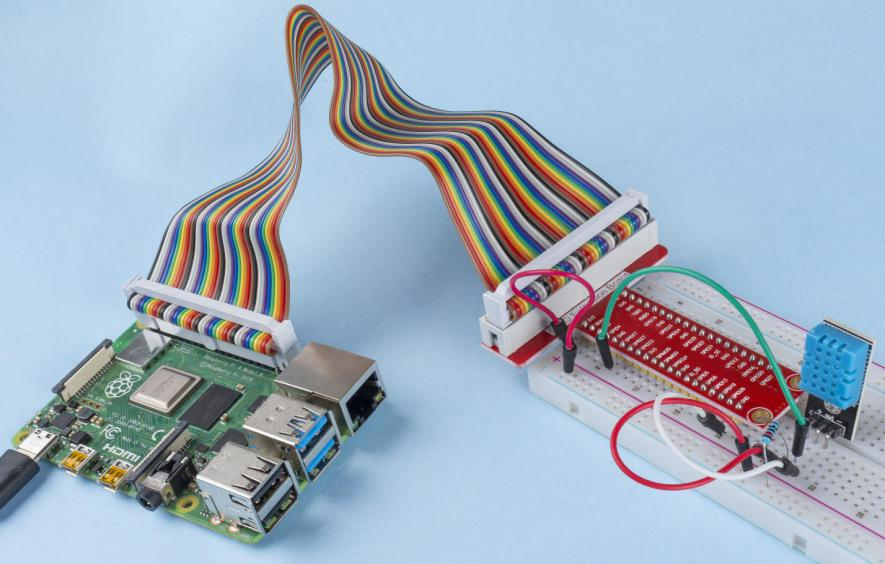# 2.2.3 DHT-11¶

## Introduction¶

The digital temperature and humidity sensor DHT11 is a composite sensor that contains a calibrated digital signal output of temperature and humidity. The technology of a dedicated digital modules collection and the technology of the temperature and humidity sensing are applied to ensure that the product has high reliability and excellent stability.

The sensors include a wet element resistive sensor and a NTC temperature sensor and they are connected to a high performance 8-bit microcontroller.

## Components¶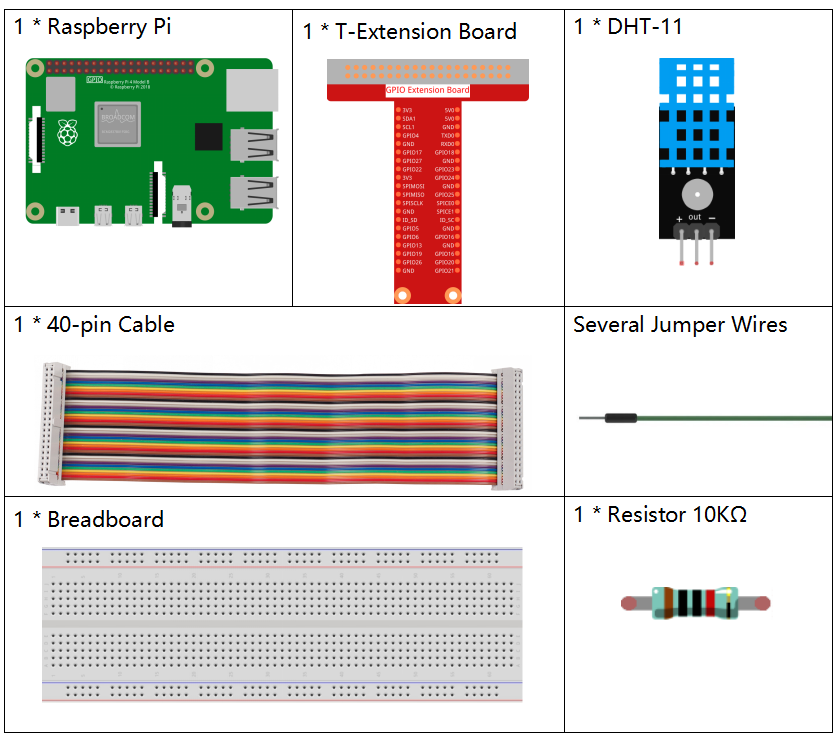## Principle¶

The DHT11 is a basic, ultra low-cost digital temperature and humidity sensor. It uses a capacitive humidity sensor and a thermistor to measure the surrounding air, and spits out a digital signal on the data pin (no analog input pins are needed).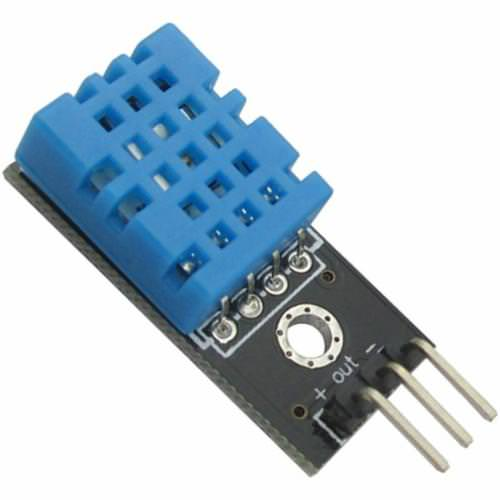Only three pins are available: VCC, GND, and DATA. The communication process begins with the DATA line sending start signals to DHT11, and DHT11 receives the signals and returns an answer signal. Then the host receives the answer signal and begins to receive 40-bit humiture data (8-bit humidity integer + 8-bit humidity decimal + 8-bit temperature integer + 8-bit temperature decimal + 8-bit checksum). For more information, please refer to DHT11 datasheet.

## Schematic Diagram¶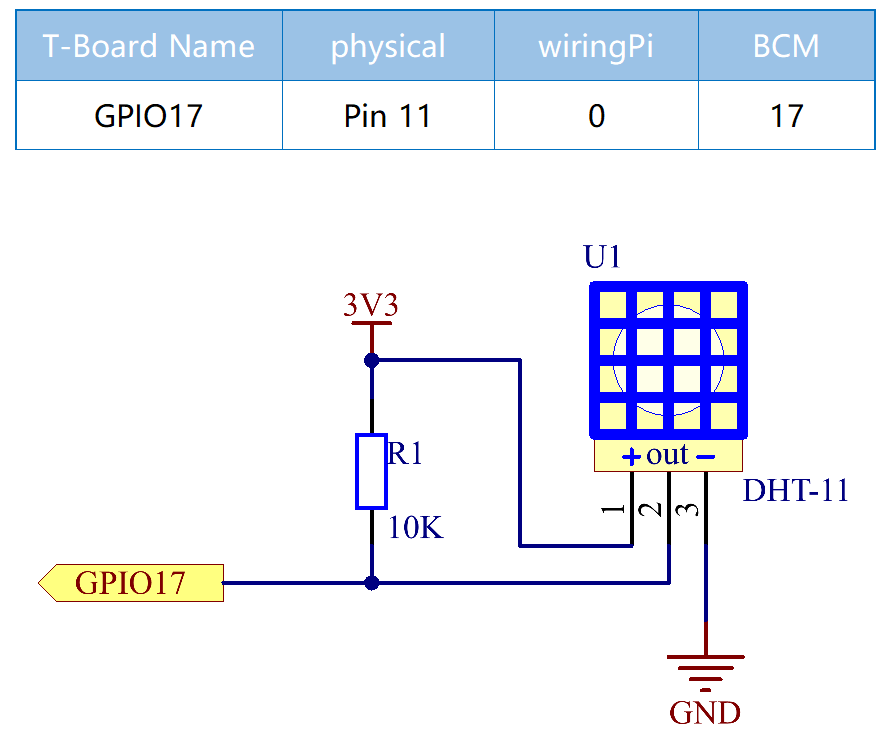## Experimental Procedures¶

Step 1: Build the circuit.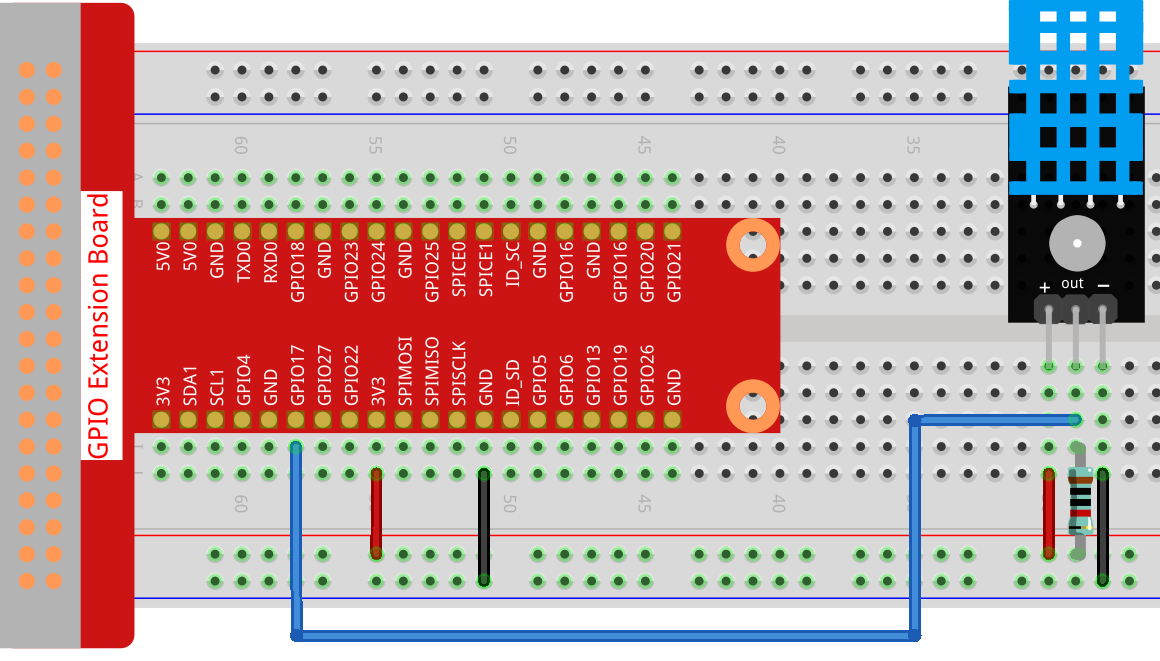### For C Language Users¶

Step 2: Go to the folder of the code.

```cd ~/davinci-kit-for-raspberry-pi/c/2.2.3/
```

Step 3: Compile the code.

```gcc 2.2.3_DHT.c -lwiringPi
```

Step 4: Run the executable file.

```sudo ./a.out
```

After the code runs, the program will print the temperature and humidity detected by DHT11 on the computer screen.

Note

If it does not work after running, or there is an error prompt: "wiringPi.h: No such file or directory", please refer to C code is not working?.

Code

```#include <wiringPi.h>
#include <stdio.h>
#include <stdlib.h>
#include <stdint.h>

#define maxTim 85
#define dhtPin 0

int dht11_dat = {0,0,0,0,0};

uint8_t laststate = HIGH;
uint8_t counter = 0;
uint8_t j = 0, i;
float Fah; // fahrenheit
dht11_dat = dht11_dat = dht11_dat = dht11_dat = dht11_dat = 0;
// pull pin down for 18 milliseconds
pinMode(dhtPin, OUTPUT);
digitalWrite(dhtPin, LOW);
delay(18);
// then pull it up for 40 microseconds
digitalWrite(dhtPin, HIGH);
delayMicroseconds(40);
// prepare to read the pin
pinMode(dhtPin, INPUT);

// detect change and read data
for ( i=0; i< maxTim; i++) {
counter = 0;
counter++;
delayMicroseconds(1);
if (counter == 255) {
break;
}
}

if (counter == 255) break;
// ignore first 3 transitions
if ((i >= 4) && (i%2 == 0)) {
// shove each bit into the storage bytes
dht11_dat[j/8] <<= 1;
if (counter > 50)
dht11_dat[j/8] |= 1;
j++;
}
}
// check we read 40 bits (8bit x 5 ) + verify checksum in the last byte
// print it out if data is good
if ((j >= 40) &&
(dht11_dat == ((dht11_dat + dht11_dat + dht11_dat + dht11_dat) & 0xFF)) ) {
Fah = dht11_dat * 9. / 5. + 32;
printf("Humidity = %d.%d %% Temperature = %d.%d *C (%.1f *F)\n",
dht11_dat, dht11_dat, dht11_dat, dht11_dat, Fah);
}
}

int main (void) {
if(wiringPiSetup() == -1){ //when initialize wiring failed, print messageto screen
printf("setup wiringPi failed !");
return 1;
}
while (1) {
delay(500); // wait 1sec to refresh
}
return 0 ;
}
```

Code Explanation

```void readDht11() {
uint8_t laststate = HIGH;
uint8_t counter = 0;
uint8_t j = 0, i;
float Fah; // fahrenheit
dht11_dat = dht11_dat = dht11_dat = dht11_dat = dht11_dat = 0;
// ...
}
```

This function is used to realize the function of DHT11.

It generally can be divided into 3 parts:

1. prepare to read the pin:

```// pull pin down for 18 milliseconds
pinMode(dhtPin, OUTPUT);
digitalWrite(dhtPin, LOW);
delay(18);
// then pull it up for 40 microseconds
digitalWrite(dhtPin, HIGH);
delayMicroseconds(40);
// prepare to read the pin
pinMode(dhtPin, INPUT);
```

Its communication flow is determined by work timing.When DHT11 starts up, MCU will send a low level signal and then keep the signal at high level for 40us. After that, the detection of the condition of external environment will start.

```// detect change and read data
for ( i=0; i< maxTim; i++) {
counter = 0;
counter++;
delayMicroseconds(1);
if (counter == 255) {
break;
}
}
if (counter == 255) break;
// ignore first 3 transitions
if ((i >= 4) && (i%2 == 0)) {
// shove each bit into the storage bytes
dht11_dat[j/8] <<= 1;
if (counter > 50)
dht11_dat[j/8] |= 1;
j++;
}
}
```

The loop stores the detected data in the dht11_dat[] array. DHT11 transmits data of 40 bits at a time. The first 16 bits are related to humidity, the middle 16 bits are related to temperature, and the last eight bits are used for verification. The data format is:

8bit humidity integer data + 8bit humidity decimal data + 8bit temperature integer data + 8bit temperature decimal data + 8bit check bit.

1. Print Humidity & Temperature.

```// check we read 40 bits (8bit x 5 ) + verify checksum in the last byte
// print it out if data is good
if ((j >= 40) &&
(dht11_dat == ((dht11_dat + dht11_dat + dht11_dat + dht11_dat) & 0xFF)) ) {
Fah = dht11_dat * 9. / 5. + 32;
printf("Humidity = %d.%d %% Temperature = %d.%d *C (%.1f *F)\n",
dht11_dat, dht11_dat, dht11_dat, dht11_dat, Fah);
}
```

When the data storage is up to 40 bits, check the validity of the data through the check bit (dht11_dat), and then print the temperature and humidity.

For example, if the received data is 00101011(8-bit value of humidity integer) 00000000 (8-bit value of humidity decimal) 00111100 (8-bit value of temperature integer) 00000000 (8-bit value of temperature decimal) 01100111 (check bit)

Calculation:

00101011+00000000+00111100+00000000=01100111.

The final result is equal to the check bit data, then the received data is correct:

Humidity =43%，Temperature =60*C.

If it is not equal to the check bit data, the data transmission is not normal and the data is received again.

### For Python Language Users¶

Step 2: Go to the folder of the code.

```cd ~/davinci-kit-for-raspberry-pi/python/
```

Step 3: Run the executable file.

```sudo python3 2.2.3_DHT.py
```

After the code runs, the program will print the temperature and humidity detected by DHT11 on the computer screen.

Code

Note

You can Modify/Reset/Copy/Run/Stop the code below. But before that, you need to go to source code path like `davinci-kit-for-raspberry-pi/python`.

```import RPi.GPIO as GPIO
import time

dhtPin = 17

GPIO.setmode(GPIO.BCM)

MAX_UNCHANGE_COUNT = 100

STATE_INIT_PULL_DOWN = 1
STATE_INIT_PULL_UP = 2
STATE_DATA_FIRST_PULL_DOWN = 3
STATE_DATA_PULL_UP = 4
STATE_DATA_PULL_DOWN = 5

GPIO.setup(dhtPin, GPIO.OUT)
GPIO.output(dhtPin, GPIO.HIGH)
time.sleep(0.05)
GPIO.output(dhtPin, GPIO.LOW)
time.sleep(0.02)
GPIO.setup(dhtPin, GPIO.IN, GPIO.PUD_UP)

unchanged_count = 0
last = -1
data = []
while True:
current = GPIO.input(dhtPin)
data.append(current)
if last != current:
unchanged_count = 0
last = current
else:
unchanged_count += 1
if unchanged_count > MAX_UNCHANGE_COUNT:
break

state = STATE_INIT_PULL_DOWN

lengths = []
current_length = 0

for current in data:
current_length += 1

if state == STATE_INIT_PULL_DOWN:
if current == GPIO.LOW:
state = STATE_INIT_PULL_UP
else:
continue
if state == STATE_INIT_PULL_UP:
if current == GPIO.HIGH:
state = STATE_DATA_FIRST_PULL_DOWN
else:
continue
if state == STATE_DATA_FIRST_PULL_DOWN:
if current == GPIO.LOW:
state = STATE_DATA_PULL_UP
else:
continue
if state == STATE_DATA_PULL_UP:
if current == GPIO.HIGH:
current_length = 0
state = STATE_DATA_PULL_DOWN
else:
continue
if state == STATE_DATA_PULL_DOWN:
if current == GPIO.LOW:
lengths.append(current_length)
state = STATE_DATA_PULL_UP
else:
continue
if len(lengths) != 40:
#print ("Data not good, skip")
return False

shortest_pull_up = min(lengths)
longest_pull_up = max(lengths)
halfway = (longest_pull_up + shortest_pull_up) / 2
bits = []
the_bytes = []
byte = 0

for length in lengths:
bit = 0
if length > halfway:
bit = 1
bits.append(bit)
#print ("bits: %s, length: %d" % (bits, len(bits)))
for i in range(0, len(bits)):
byte = byte << 1
if (bits[i]):
byte = byte | 1
else:
byte = byte | 0
if ((i + 1) % 8 == 0):
the_bytes.append(byte)
byte = 0
#print (the_bytes)
checksum = (the_bytes + the_bytes + the_bytes + the_bytes) & 0xFF
if the_bytes != checksum:
#print ("Data not good, skip")
return False

return the_bytes, the_bytes

def main():

while True:
if result:
humidity, temperature = result
print ("humidity: %s %%,  Temperature: %s C`" % (humidity, temperature))
time.sleep(1)

def destroy():
GPIO.cleanup()

if __name__ == '__main__':
try:
main()
except KeyboardInterrupt:
destroy()
```

Code Explanation

```def readDht11():
GPIO.setup(dhtPin, GPIO.OUT)
GPIO.output(dhtPin, GPIO.HIGH)
time.sleep(0.05)
GPIO.output(dhtPin, GPIO.LOW)
time.sleep(0.02)
GPIO.setup(dhtPin, GPIO.IN, GPIO.PUD_UP)
unchanged_count = 0
last = -1
data = []
#...
```

This function is used to implement the functions of DHT11. It stores the detected data in the the_bytes[] array. DHT11 transmits data of 40 bits at a time. The first 16 bits are related to humidity, the middle 16 bits are related to temperature, and the last eight bits are used for verification. The data format is:

8bit humidity integer data +8bit humidity decimal data +8bit temperature integer data + 8bit temperature decimal data + 8bit check bit.

When the validity is detected via the check bit, the function returns two results: 1. error; 2. humidity and temperature.

```checksum = (the_bytes + the_bytes + the_bytes + the_bytes) & 0xFF
if the_bytes != checksum:
#print ("Data not good, skip")
return False

return the_bytes, the_bytes
```

For example, if the received date is 00101011(8-bit value of humidity integer) 00000000 (8-bit value of humidity decimal) 00111100 (8-bit value of temperature integer) 00000000 (8-bit value of temperature decimal) 01100111 (check bit)

Calculation:

00101011+00000000+00111100+00000000=01100111.

If the final result is equal to the check bit data, the data transmission is abnormal: return False.

If the final result is equal to the check bit data, the received data is correct, then there will return the_bytes and the_bytes and output "Humidity =43%，Temperature =60C".

## Phenomenon Picture¶